# CBSE Question Paper 2005 class 12 Chemistry## myCBSEguide App

Complete Guide for CBSE Students

NCERT Solutions, NCERT Exemplars, Revison Notes, Free Videos, CBSE Papers, MCQ Tests & more.

CBSE Question Paper 2005 class 12 Chemistry conducted by Central Board of Secondary Education, New Delhi in the month of March 2005. CBSE previous year question papers with the solution are available in the myCBSEguide mobile app and website. The Best CBSE App for students and teachers is myCBSEguide which provides complete study material and practice papers to CBSE schools in India and abroad.

CBSE Question Paper 2005 class 12 Chemistry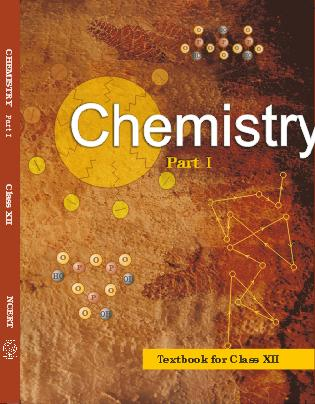## Class 12 Chemistry list of chapters

1. The Solid State
2. Solutions
3. Electrochemistry
4. Chemical Kinetics
5. Surface Chemistry
6. General Principles and Processes of Isolation of Elements
7. The p-Block Elements
8. The d and f Block Elements
9. Coordination Compounds
10. Haloalkanes and Haloarenes
11. Alcohols, Phenols and Ethers
12. Aldehydes, Ketones and Carboxylic Acids
13. Amines
14. Biomolecules
15. Polymers
16. Chemistry in Everyday life

## CBSE Question Paper 2005 class 12 Chemistry

General Instructions

1. All questions are compulsory.
2. Marks for each question are indicated against it.
3. Question numbers 1 to 5 are very short-answer questions, carrying 1 mark each. Answer these in one word or about one sentence each.
4. Question numbers 6 to 12 are short-answer questions, carrying 2 marks each. Answer these in about 30 words each.
5. Question numbers 13 to 24 are short-answer questions of 3 marks each. Answer these in about 40 words each.
6. Question numbers 25 to 27 are long-answer questions of 5 marks each. Answer these in about 70 words each.
7. Use Log Tables, if necessary Use of calculators is not permitted.

1. How many atoms can be assigned to its unit cell if an element forms (i) body centred cubic cell, and (ii) a face centred cubic cell? 

2. What would be the value of Van’t Hoff factor for a dilute solution of K2SO4 in water? 

3. Express the relation between the half-life periodof a reactant and its initial concentration for a reaction of nth order. 

4. Mention a chemical property in which methanoic acid differs from acetic acid. 

5. How is the basic strength of aromatic amines affected by the presence of an electron releasing group on the benzene ring? 

6. State that de Broglie relationship. How do de Broglie waves of a moving particle differ from electromagnetic waves? 

Or

Show that the uncertainty principle is of little significance for an object of mass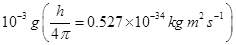7. Predict the products of electrolysis obtained at the electrodes in each case when the electrodes used are of platinum: 

(i) An aqueous solution of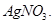(ii) An aqueous solution of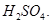8. State the basic reason of each of the following statements: 

(i) In CI undergoes disproportionate reaction but TICI does not.

(ii) AICI3 acts as a Lewis acid.

9. Write chemical equations for the following reactions: 

(i)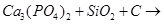(ii)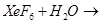10. Identify and mark the presence of centres of chirality’s, if any, in the following molecules.
Mention the number of stereoisomer possible in each case.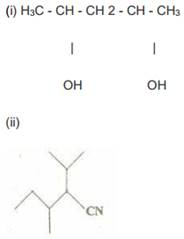11. Explain how an OH group attached to a carbon in the benzene ring activates benzene towards electrophilic substitution. 

12. How are polymers classified on the basis of forces operating between their molecules? To which, of these classes does nylon-66 belong? 

13. (a) Use the LCAO method for the formation of molecular orbitals in case of homonuclear diatomic hydrogen molecule.

(b) Which of the following has higher bond dissociation energy and why?

(i)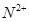(ii)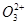Or

What kinds of molecular forces exist between the species in the following pairs of particles and why?

(i) He and N2

(ii) Cl2 and NO3-

(iii) NH3 and CO 

14. Aluminum crystallizes in a face centred cubic close-packed structure its atomic radius is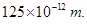(a) What is the length of the edge of the unit cell?

(b) How many such unit cells are there in a 1.00 m piece of aluminium? 

15. State Henry’s law for solubility of a gas in a liquid Explain the significance of Henry’s law constant ( At the same temperature, hydrogen Is more soluble in water than helium. Which of them will have a higher value of KH and why? 

16. The activation energy of a reaction is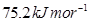in the absence of a catalyst and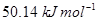with a catalyst. How many times will the rate of reaction grow in the presence of the catalyst if the reaction proceeds at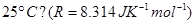17. How do size of particles of adsorbent, pressure of gas and prevailing temperature influence the extent of adsorption of a gas on a solid? 

I8. (a) Write the structural formula of hex-2-en-4ynoic acid.

(b) To illustrate the following reactions write one chemical equation for each:

(i) Cross aldol condensation

(ii) Hofmann bromamide reaction 

19. Write the chemical reaction equation stating the reaction conditions required for each of the following conversions: 

(i) Methyl bromide to ethylamine

(ii) Aniline to phenol

(iii) ptoluidine to 2-bromo-4-methylanilline

20. (a) Write the corresponding chemical reaction equation to show that

(i) PbO2 can act as an oxidizing agent.

(ii) All the bonds in a molecule of PCI5 are not equivalent.

(b) Write the structural formula for either XeF2 or IF3. 

21. Draw a sketch to show the splitting of d-orbital in an octahedral crystal field. State clearly how the actual configuration in split d-orbital in an octahedral crystal field is decided by the magnitudes of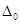and P values. 

22. The E° values at 298 K corresponding to the following two reduction electrode processes are: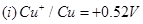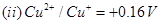Formulate the galvanic cell for their combination. What will be the cell potential?

Calculate the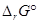for the cell reaction.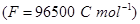23. The radioactive isotope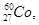can be made by an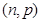or an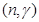nuclear reaction. State the appropriate target nucleus for each reaction. If the half-life of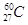is 7 years, how long will it take for complete annihilation and why? 

24. Describe the following with an example each: 

(i) Antimicrobials

(ii) Acid dyes

(iii) Antioxidants

25. (a) The standard Gibbs energy change values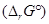at 1773 K are given for the following reactions: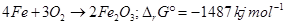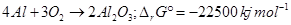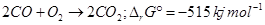Find out the possibility of reducing Fe2O3 and Al2O3 with CO at this temperature.

(b) Comment on the following statements giving reasons:

(i) An exothermic reaction is sometimes not spontaneous.

(ii) Reactions withvalues less than zero always have equilibrium constants greater than 1. 

Or

(a) The half-reactions are:

(i)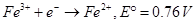(ii)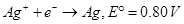Calculate Kc for the following reaction at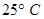: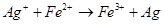(F=96500 C mol-1)

(b) Define the following terms:

(ii) State variables / State functions 

26. (a) Given below are the electrode potential values,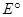for some of the first row of transition elements:

 Element V(23) Cr(24) Mn(25) Fe(26) Co(27) Ni(28) Cu(29)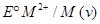-1.18 -0.91 -1.18 -0.44 -0.28 -0.25 +0.34

Explain the irregularities in these values on the basis of electronic structures of atoms.

(b) Complete the following reaction equations: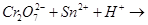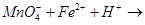Or

(a) How would you account for the following:
(i) Cobalt (II) is stable in aqueous solution. but in the presence of complexing reagents it is easily oxidized.
(ii) The transition elements exhibit high enthalpy of atomization.
(iii) Of the d4 species, Cr2+ is strongly reducing while Mn (III) is strongly oxidizing.

(b) Name the chief ore of copper and write the reactions Involved in its extraction from that ore. 

27. (a) Write the chemical reactions of glucose with (i)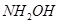and (ii)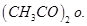Also draw simple Fischer projections of D-glucose and L-glucose.

(b) Name the food sources and the deficiency diseases caused due to lack of any two of vitamins A, C, E and K. 

Or

(a) State the composition and functional differences between DNA and RNA.
Describe the mechanism of replication of DNA.

(b) Define ‘mutation’. [3, 2]

These are questions only. To view and download complete question paper with solution install myCBSEguide App from google play store or login to our student dashboard.

## Chemistry Question Paper 2008

Download class 12 Chemistry question paper with solution from best CBSE App the myCBSEguide. CBSE class 12 Chemistry question paper 2008 in PDF format with solution will help you to understand the latest question paper pattern and marking scheme of the CBSE board examination. You will get to know the difficulty level of the question paper. CBSE question papers 2008 for class 12 Chemistry have 27 questions with solution.

## Previous Year Question Paper for class 12 in PDF

CBSE question papers 2018, 2017, 2016, 2015, 2014, 2013, 2012, 2011, 2010, 209, 2008, 2007, 2006, 2005 and so on for all the subjects are available under this download link. Practicing real question paper certainly helps students to get confidence and improve performance in weak areas.

To download CBSE Question Paper 2008 class 12 Accountancy, Chemistry, Physics, History, Political Science, Economics, Geography, Computer Science, Home Science, Accountancy, Business Studies and Home Science; do check myCBSEguide app or website. myCBSEguide provides sample papers with solution, test papers for chapter-wise practice, NCERT solutions, NCERT Exemplar solutions, quick revision notes for ready reference, CBSE guess papers and CBSE important question papers. Sample Paper all are made available through the best app for CBSE students and myCBSEguide website.## Test Generator

Create Papers with your Name & Logo